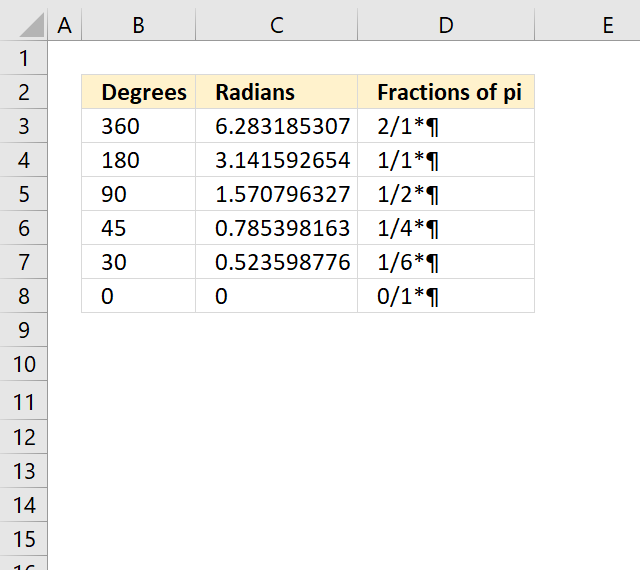Author: Oscar Cronquist Article last updated on October 27, 2022The formula in cell D3 converts the radian decimal value to fractions of pi.

Formula in cell D3:

=TEXT(C3/PI(),"?/?")&"*"&CHAR(182)

### Explaining the formula in cell D3

#### Step 1 - Divide radian by pi

The first operation is the division between the radian number and pi.

C3/PI()

becomes

6.28318530717959/PI()

The PI function returns the number pi (¶).

Function syntax: PI()

6.28318530717959/PI()

becomes

6.28318530717959/3.14159265358979

and returns 2

#### Step 2 - Calculate fractions

The TEXT function converts a value to text in a specific number format.

Function syntax: TEXT(value, format_text)

TEXT(C3/PI(),"?/?")

becomes

TEXT(2,"?/?")

and returns "2/1".

#### Step 3 - Display the PI character

The CHAR function converts a number to the corresponding ANSI character determined by your computers character set.

Function syntax: CHAR(text)

The CHAR function inserts the pi symbol using ANSI code 182.

CHAR(182)

returns ¶

#### Step 4 - Join values

Lastly, the ampersands concatenate the values.

TEXT(C3/PI(),"?/?")&"*"&CHAR(182)

becomes

"2/1"&"*"&¶

and returns "2/1*¶".

### Get Excel *.xlsx file

Convert radians to fractions of pi.xlsx

Recommended articles

How to use the RADIANS function

Recommended articles

How to use the CHAR function
Use the CHAR function to convert a number to the corresponding character. This is determined by your computer's character set. […]

Recommended articles

How to use the TEXT function
This article demonstrates how to use the TEXT function in great detail. The formula in cell D3 formats the number […]

Recommended articles

How to use the PI function
The PI function returns the number pi (¶). Formula in cell B3: =PI() Excel Function Syntax PI() Arguments PI function […]